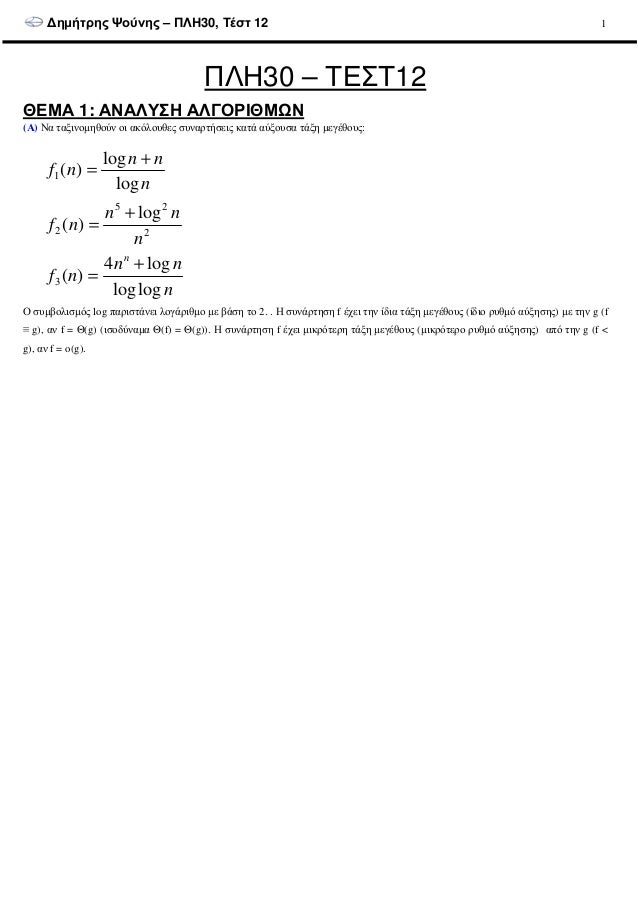Successfully reported this slideshow.
We use your LinkedIn profile and activity data to personalize ads and to show you more relevant ads. You can change your ad preferences anytime.Upcoming SlideShare
×

# ΠΛΗ30 ΤΕΣΤ 12

1,178 views

Published on

1.1) Ιεραρχία Συναρτήσεων Πολυπλοκότητας
1.2) Αναδρομικές Σχέσεις (Θεώρημα Κυριαρχίας, Μέθοδος Επανάληψης)
3.1) ΚΕ σε ΜΠΑε
3.2) "αρχίζει" και "περιέχει" και "τελειώνει": ΚΕ - ΜΠΑ - ΝΠΑ

Published in: Education
• Full Name
Comment goes here.

Are you sure you want to Yes No
Your message goes here• Be the first to comment

• Be the first to like this

### ΠΛΗ30 ΤΕΣΤ 12

1. 1. ∆ηµήτρης Ψούνης – ΠΛΗ30, Τέστ 12 1 ΠΛΗ30 – ΤΕΣΤ12 ΘΕΜΑ 1: ΑΝΑΛΥΣΗ ΑΛΓΟΡΙΘΜΩΝ (Α) Να ταξινοµηθούν οι ακόλουθες συναρτήσεις κατά αύξουσα τάξη µεγέθους: n nn nf n nn nf n nn nf n loglog log4 )( log )( log log )( 3 2 25 2 1 + = + = + = Ο συµβολισµός log παριστάνει λογάριθµο µε βάση το 2. . Η συνάρτηση f έχει την ίδια τάξη µεγέθους (ίδιο ρυθµό αύξησης) µε την g (f ≡ g), αν f = Θ(g) (ισοδύναµα Θ(f) = Θ(g)). Η συνάρτηση f έχει µικρότερη τάξη µεγέθους (µικρότερο ρυθµό αύξησης) από την g (f < g), αν f = o(g).
2. 2. ∆ηµήτρης Ψούνης – ΠΛΗ30, Τέστ 12 2 (Β) Να λύσετε τις αναδροµές: 2 85 4 )()1( n n T n TnT +      +      = 2 4 23)()2( n n TnT +      = n n TnT +      = 9 3)()3( ( ) 10 41)()4( nnTnT +−= Στη συνέχεια, να διαταχθούν οι λύσεις τους κατά αύξουσα τάξη µεγέθους. Θεώρηµα Κυριαρχίας: Έστω η αναδροµική εξίσωση T(n) = aT(n/b) + f(n), όπου a≥1, b>1 είναι σταθερές, και f(n) είναι µια ασυµπτωτικά θετική συνάρτηση. Τότε διακρίνονται οι ακόλουθες τρεις περιπτώσεις: log log ( ) ( ), ( )b ba a (1) αν f n O n για κάποια σταθερά ε>0, τότε T(n) = nεεεε−−−− = Θ= Θ= Θ= Θ log log ( ) ( ), ( log )b ba a (2) αν f n n τότε T(n) = n n= Θ Θ= Θ Θ= Θ Θ= Θ Θ log ( ) ( ), , ( ( )). b a 0 0 (3) αν f n n για κάποια σταθερά ε>0, και αν υπάρχει σταθερά n τέτοια n ώστε, για κάθε n n , af cf(n) για κάποια σταθερά c<1, τότε T(n) = f n b εεεε++++ = Ω= Ω= Ω= Ω      ≥ ≤ Θ≥ ≤ Θ≥ ≤ Θ≥ ≤ Θ          Υπόδειξη: Θεωρείστε γνωστό ότι: )( 11 1 10 ni n i Θ=∑=
3. 3. ∆ηµήτρης Ψούνης – ΠΛΗ30, Τέστ 12 3 ΘΕΜΑ 3: ΚΑΝΟΝΙΚΕΣ ΓΛΩΣΣΕΣ Άσκηση 1: Κατασκευάστε ΜΠΑ για τις κανονικές εκφράσεις: L1 = (0+1)*11 L2 = (0+10+100+1000)* L3 = 11(0+1)* + (0+1)*001 L4 = 0*1*1* L5 = (00(0+1)*11)*
4. 4. ∆ηµήτρης Ψούνης – ΠΛΗ30, Τέστ 12 4 Άσκηση 2: ∆ίδεται η γλώσσα του αλφαβήτου {0,1} L={w|w αρχίζει µε 010, περιέχει το 110 και τελειώνει µε 111} (Α) ∆ώστε Κανονική Έκφραση που παράγει τις συµβολοσειρές της L (B) ∆ώστε Μη Ντετερµινιστικό Πεπερασµένο Αυτόµατο (ΜΠΑ) της L (Γ) ∆ώστε Ντετερµινιστικό Πεπερασµένο Αυτόµατο (ΝΠΑ) της L (∆) ∆ώστε Ντετερµινιστικό Πεπερασµένο Αυτόµατο (ΝΠΑ) για το συµπλήρωµα της L